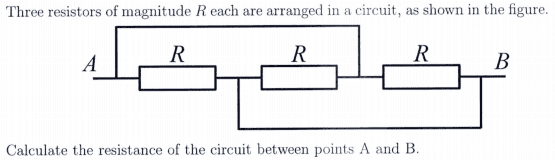# Resistors in Parallel or Series?

Homework Statement:
Three Resistors of magnitude R each are arranges in a circuit, as seen in the figure (added below).
Calculate the resistance of the circuit between points A and B.
Relevant Equations:
If they're in parallel, I have 1/R_t = 1/R + 1/R + 1/R
If they're in series, I have R_t = R + R + R

V=IRGiven that they're all on the same branch, I had assumed that they were in series with one another. But with the middle resistor having being on the middle of three branches, it looks parallel.

Like I said, I have a feeling it's in series (making the answer 3R).

This question is from a past paper that I cannot get the solutions to due to policy reasons. It's worth 15 marks out of 180, so both of these answers seem too simple.

Any help is appreciated, thanks!

phinds
Gold Member
This is a VERY widely used simple circuit that simply requires redrawing for clarification. Don't keep looking at the circuit the way it is, redraw it. That's the point of the exercise, to see how sometimes seemingly complex circuits (a bit of an exaggeration) can be simplified.

vela
Staff Emeritus
Homework Helper
You may want to clarify in your mind what exactly it means for two resistors to be in parallel or in series. When you look at that circuit, you should be able to immediately conclude that none of the resistors is in series with any of the others. (Note that this does not imply they're in parallel.)

CWatters
Homework Helper
Gold Member
I would start by working out how many nodes there are. Label them A B C etc. Also number the resistors R1 R2 etc

Then make a new drawing starting with just dots representing the nodes instead of lines/wires.

Connect the resistors between the dots. Then think about which are in parallel or series.

robphy
Homework Helper
Gold Member
In addition to labeling nodes by a letter, one can also label the nodes by color-coding each piece of wire a distinct color...
where each color represents are generally different electric potential (a different voltage reading from ground). If you cross over a circuit element, you start a new color.

Focus on connectivity (how one end of a circuit-element is connected to another circuit-element)...
not on the location of circuit-elements or on the shape of the connecting wires.

As suggested above, it might help to redraw the diagram
• circuit-elements may be repositioned and
• connecting-wires can be reshaped (even stretched out or crumpled up into a point)
• ...but do so without changing the connectivity.
As @vela suggests.... it's not "either Parallel or Series".. it's generally "Parallel, Series, or Neither".

•CWatters
kuruman